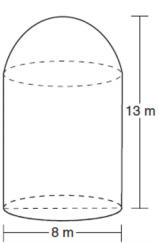mycollegehive
A storage tank is in the shape of a cylinder with a hemisphere on the top. The highest point on the inside of the storage tank is 13 meters above the floor of the storage tank, and the diameter inside the cylinder is 8 meters. Determine and state, to the nearest cubic meter, the total volume inside the storage tank.
JMAP geometry math hemisphere cylinder0A storage tank is in the shape of a cylinder with a hemisphere on the top. The highest point on the inside of the storage tank is 13 meters above the floor of the storage tank, and the diameter inside the cylinder is 8 meters. Determine and state, to the nearest cubic meter, the total volume inside the storage tank.68 viewsShareFollowUniversity of Lagos Nigeria
24 June 2020University of Benin Nigeria
05 July 20200Since the tank is a cylinder wih a hemisphere on the top, Volume of the storage tank, V = Volume of cylinder, V1 plus Volume of hemisphere, V2

That is,

V = V1 + V2

Where V1 and V2 are the volume of the cylinder and hemisphere.

V1 = πr2h

where r is the radius and h is the height of the cylinder respectively

r = diameter/2

r = 8/2 = 4

h = 13 - 4 = 9

V1 = π(4)2(9)

V1 = 452 cubic meter

V2 = 2/3 πr3

r = diameter/2

r = 8/2 = 4

V2 = 2/3 π(4)3

V2 = 134 cubic meter

Thus, V = 452 + 134

V = 586 cubic meterShare### Related Tags

JMAP

2 followers

141 questionsgeometry

2 followers

99 questionsmath

2 followers

993 questions